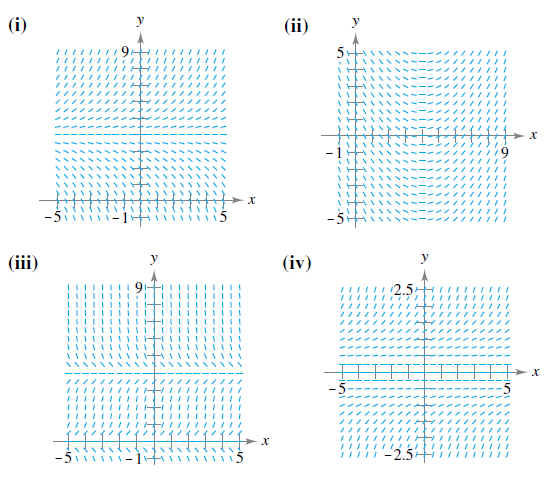Chapter 6.3, Problem 37E

Chapter
Section
Textbook Problem

Slope Field In Exercises 35-38, (a) write a differential equation for the statement, (b) match the differential equation with a possible slope field, and (c) verify your result by using a graphing utility to graph a slope field for the differential equation. [The slope fields are labeled (i), (ii), (iii), and (iv).]The rate of change of y with respect to x is proportional to the product of y and the difference between y and 4.

(a)

To determine

To Calculate: The differential equation of the statement, “The rate of change of y with respect to x is proportional to the product y and the difference between y and 4”.

Explanation

Given:

The statement, “The rate of change of y with respect to x is proportional to the product y and the difference between y and 4”.

Formula used:

The rate of change of y with respect to x is given by dydx.

Calculation:

Let us consider the given differential is as follows:

dydxα

(b)

To determine
The correct match of the differential equation dydx=ky(y4).

(c)

To determine

To prove: The result by using graphing utility to graph a slope field for the differential equation dydx=yk(y4) .

Still sussing out bartleby?

Check out a sample textbook solution.

See a sample solution

The Solution to Your Study Problems

Bartleby provides explanations to thousands of textbook problems written by our experts, many with advanced degrees!

Get Started

Find more solutions based on key concepts